Open In App
Related Articles

# Concave and Convex Mirrors

Mirrors are surfaces that reflect almost all types of incident light rays that hit their surface. The mirror can have either a plane or a curved surface. On this basis, mirrors are divided into two: Spherical Mirrors and Plane Mirrors. Plane mirrors simply mirror having plain and polished reflecting surfaces while Spherical mirrors have curved reflecting surfaces. Based on the curved reflecting surface spherical mirrors are further divided into two: Concave and Convex Mirrors

In concave mirrors, the reflection takes place from the inner surface of the spherical mirror. The image formed by the concave mirrors is real and inverted. However, in the case of convex mirrors, the reflection takes place from the outer surface of the spherical mirror. The image formed by the convex mirrors is virtual and erect.

## What are Mirrors?

A mirror is a reflective surface that reflects light and creates a real or imaginary image.

When an object is placed in front of a mirror, the mirror reflects the image of the same object. The incident rays are coming from the object, and the reflected rays are what produce the image. The classification of the images as real or virtual depends on where the light rays intersect.

The two types of mirrors that are most commonly used are:

• Spherical Mirrors
• Plane Mirrors

## Plane Mirrors

The most typical mirrors are flat and are referred to as “plane mirrors.” A fine layer of silver nitrate or aluminum is deposited behind a flat piece of glass to construct plane mirrors.

When a light beam strikes a plane mirror, the light ray is reflected in such a way that it makes an equal angle with the incident ray that is, the angle of reflection is equal to the angle of incidence.

## Spherical Mirrors

The curved surface we see of a shining spoon can be considered a curved mirror. The most widely used type of curved mirror is the spherical mirror.

The reflecting surface of such mirrors is considered to form a part of the surface of any sphere. Those mirrors which possess reflecting surfaces which are spherical are called spherical mirrors.

## Important Terms Used in Spherical Mirrors

• Pole: The midpoint or the center point of the spherical mirror. It is represented by capital P. All the measurements are made from it only.
• Aperture: An aperture of a mirror is a point from which the reflection of light actually takes place or happens. It also gives an idea about the size of the mirror.
• Principal axis: An imaginary line that passes through the optical center and from the center of curvature of a spherical mirror. All the measurements are based on this line.
• Centre of Curvature: The point in the center of the mirror surface that passes through the curve of the mirror and has the same tangent and curvature at that point. It is represented by the capital letter C.
• Radius of Curvature: It is considered as the linear distance between the pole and the center of curvature. It is represented by the capital letter R,
• Principal Focus: Principal Focus can be called the Focal Point also. It is present on the axis of a mirror where the rays of light parallel to the principal axis converge or appear to converge or diverge after reflection.
• Focus: It is any given point on the principal axis where light rays parallel to the principal axis will converge or appear to converge after getting reflected from the mirror.

## Types of Spherical Mirrors

Spherical Mirrors are categorized into two types:

• Concave Mirrors
• Convex Mirrors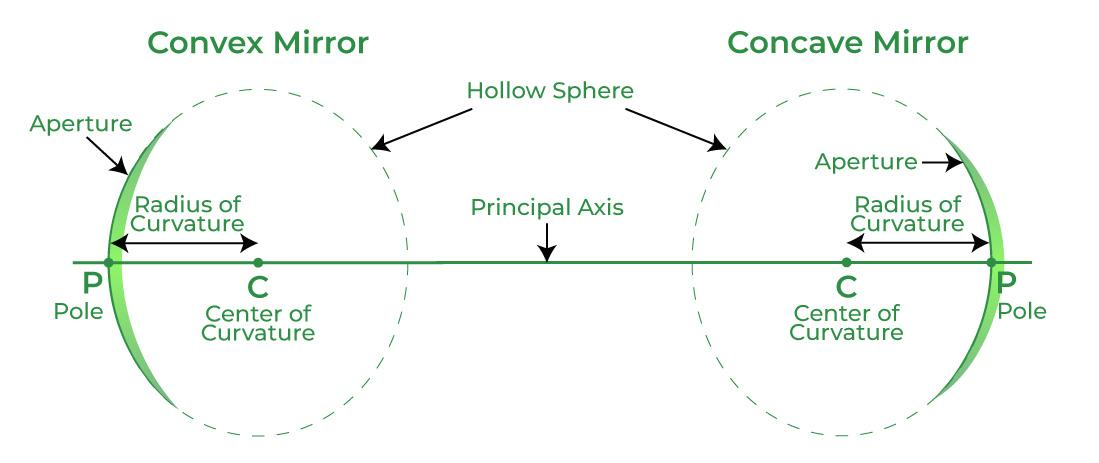Types of Spherical Mirrors

## Concave Mirror

If a hollow sphere is cut into some parts and the outer surface of the cut part is painted, then it turns out to be a mirror with its inner surface as the reflecting surface. This makes a concave mirror.

A concave mirror or converging mirror is a type of mirror that is bent towards the inwards side in the middle. Moreover, by looking in this mirror, we will feel that we are looking in a cave. We tend to use the mirror equation to deal with a concave mirror.

The equation for these mirrors determines the position of the object and the accurate size of the object. The angle of incidence in the concave mirror is not the same as the angle of reflection. Moreover, the angle of reflection, in this case, depends on the area on which the light hits.

### Properties of Concave Mirrors

• Light after reflection converges at a point when it strikes and reflects back from the reflecting surface of the concave mirror. Hence, it is also termed a converging mirror.
• When the converging mirror is placed very near to the object, a magnified and virtual image is observed.
• But, if we tend to increase the distance between the object and the mirror, then the image’s size reduces, and a real image is formed.
• The image formed by the concave mirror can be small or enlarged or can be either real or virtual.

### Applications of Concave Mirrors

• Used in shaving mirrors: Converging mirrors are most widely used in shaving because they have reflective and curved surfaces. At the time of shaving, the concave mirror forms an enlarged as well as erect image of the face when the concave mirror is held closer to the face.
• The concave mirror used in the ophthalmoscope: These mirrors are used in optical instruments as in ophthalmoscopes for treatment.
• Uses of the concave mirrors in astronomical telescopes: These mirrors are also widely used in making astronomical telescopes. In an astronomical telescope, a converging mirror of a diameter of about 5 meters or more is used as the objective.
• Concave mirrors used in the headlights of vehicles: Converging mirrors are widely used in the headlights of automobiles and in motor vehicles, torchlights, railway engines, etc. as reflectors. The point light source is kept at the focus of the mirror, so after reflection, the light rays travel over a huge distance as parallel light beams of high intensity.
• Used in solar furnaces: Large converging mirrors are used to focus the sunlight to produce heat in the solar furnace. They are often used in solar ovens to gather a large amount of solar energy in the focus of the concave mirror for heating, cooking, melting metals, etc.

## Image Formation by Concave Mirror and their ray diagrams

When the object is placed at infinity

As the parallel rays from the object converge at the principal focus, F of a concave mirror; after reflection through it. Therefore, when the object is at infinity the image will form at F.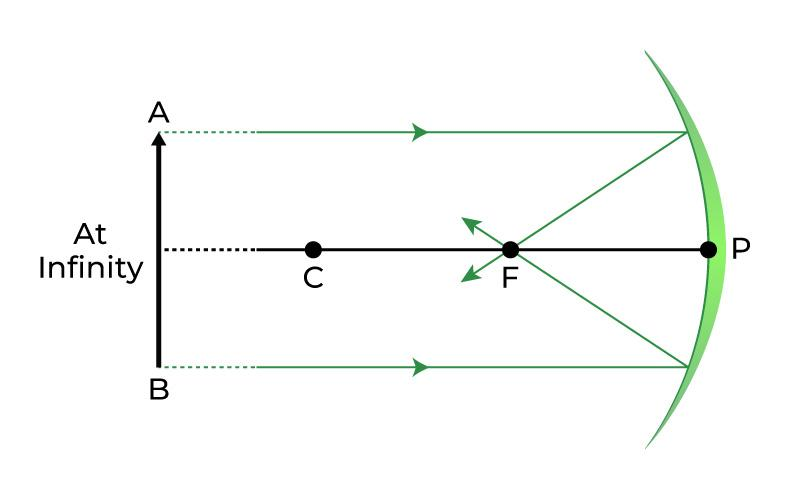Properties of the image formed: Point-sized image, highly diminished in size, Real and inverted image.

When the object is placed between infinity and the Centre of Curvature

When the object is placed between infinity and the center of curvature of a concave mirror then the image is formed between the center of curvature (C) and focus (F).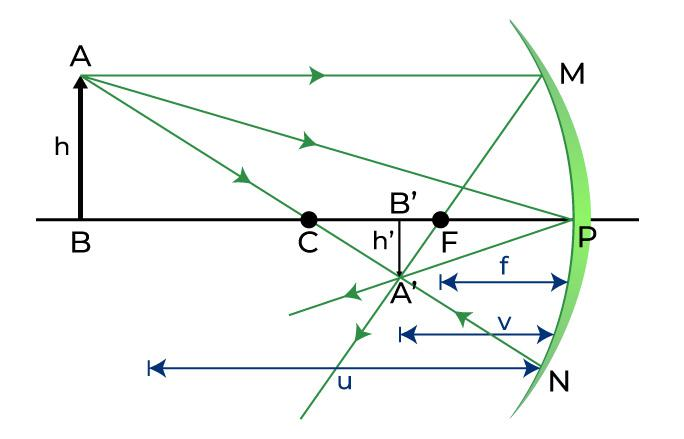Properties of image: It is diminished as compared to the object and also real and inverted.

Object at Centre of Curvature (C)

Whenever we place our object at the center of curvature (C) of a concave mirror, we get a real and inverted image formed at the same position.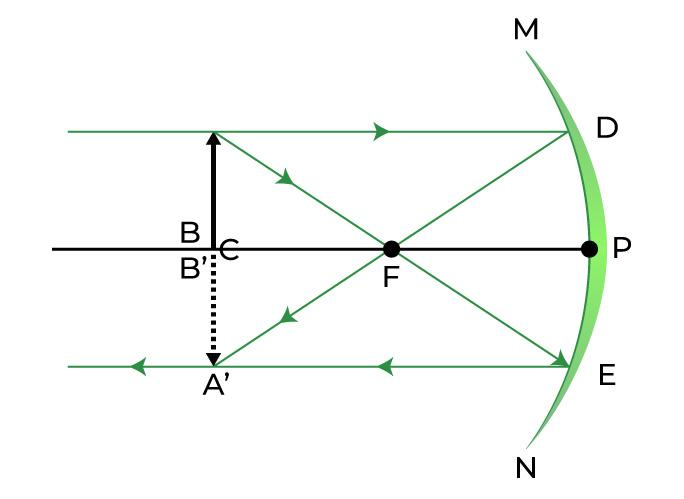Properties of image: It is of the same size as the object and also real and inverted.

The object is kept between the Centre of curvature (C) and Principal Focus (F)

When we keep the object somewhere between the center of curvature and the principal focus of the concave mirror, a real image is formed placed beyond the center of curvature (C).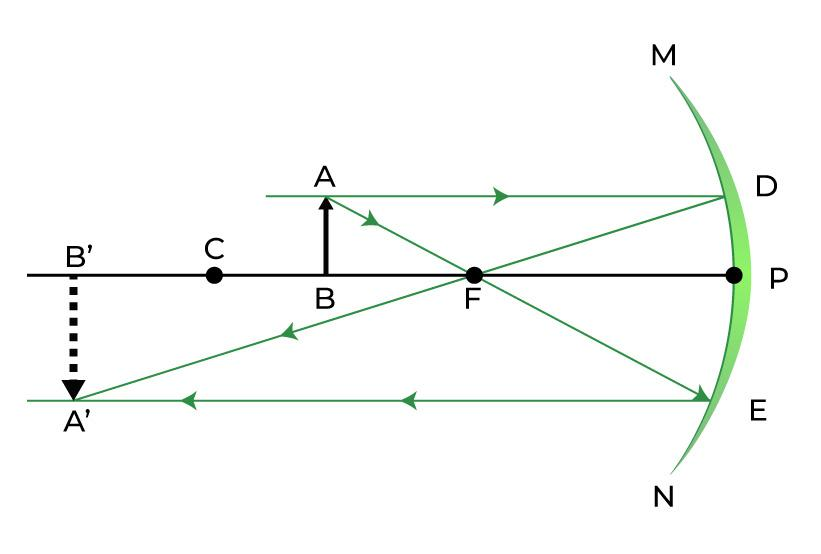Object at Principal Focus (F)

When the object is placed at the principal focus (F) of a concave mirror, a highly enlarged image of the object is formed at infinity.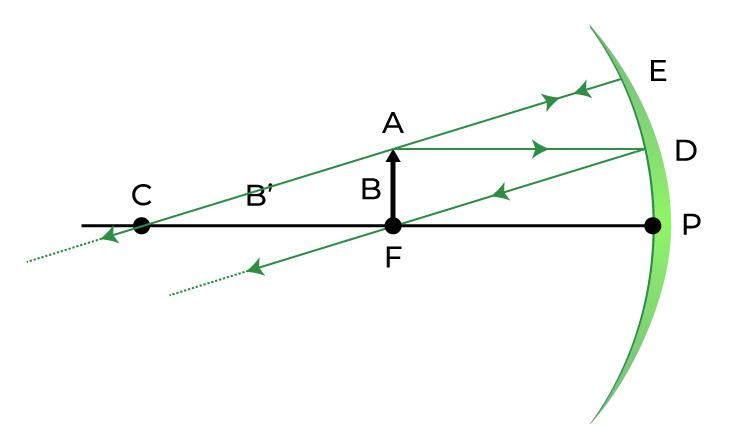Properties of image: Highly enlarged image, its nature is real and inverted

The object between Principal Focus (F) and Pole (P)

When the object is placed anywhere between the principal focus and the pole of a concave mirror, we get an enlarged, virtual and erect image formed behind the mirror.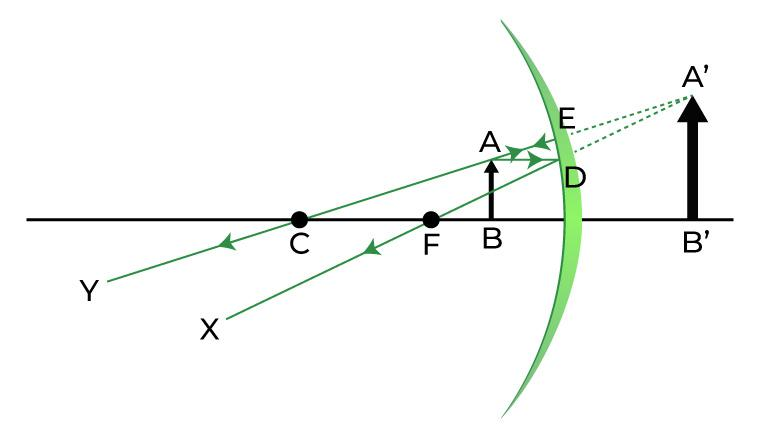Properties of image: Enlarged, Virtual and erect.

## Convex Mirror

If the cut part of the hollow sphere is painted from the inside, then its outer surface becomes the reflecting surface. This mirror is known as a convex mirror. A spherical mirror having its reflecting surface curved outwards is known to be a convex mirror.

The back of the mirror is shaded so that reflection only takes place from the outward bulged part. The surface of the spoon which bulged outwards can be assumed to be a convex mirror.

It is also known as a diverging mirror as the light after reflecting through its surface diverges in many directions but appears to meet at some points where the virtual, erect image of diminished size is formed.

### Properties of Convex Mirror

• A convex or diverging mirror is also known as a diverging mirror as this mirror diverges light when they strike its reflecting surface.
• Virtual, erect, and diminished images are always formed or observed with convex mirrors, irrespective of the distance between the object and the mirror.

### Application of Convex Mirror

• Convex mirrors used inside buildings: Large offices, stores, and hospitals use a convex mirror to let people see around the corner so that they can avoid running into each other and prevent any collision.
• The convex mirrors used in vehicles: Convex mirrors are commonly used as rear-view mirrors in the case of automobiles and vehicles because they can diverge light beams and make virtual images.
• Uses of the convex mirror in a magnifying glass: These mirrors are mostly used for making magnifying glasses. In industries, to construct a magnifying glass, two convex mirrors are placed back to back.
• Convex mirrors used for security purposes: Diverging mirrors are also used for security purposes in many places. They are places near ATMs so that bank customers can check if someone is behind them.
• Convex mirrors are also used in various other places like street light reflectors as they can spread light over bigger areas.

## Image Formation by Convex Mirror and their ray diagrams

Two possibilities of the position of the object are possible in the case of a convex mirror, which is when the object is at infinity and the object is between infinity and the pole of a convex mirror.

Object at infinity

Whenever the object is kept at infinity, we observe that a point-sized image is formed at the principal focus behind the convex mirror.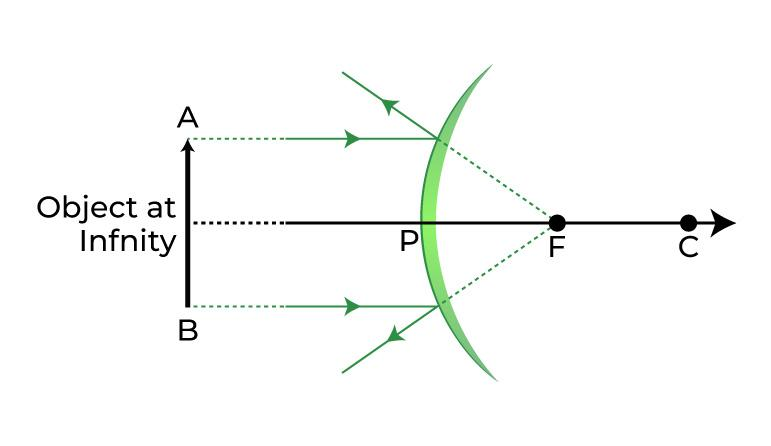Properties of image: The image formed is highly diminished in size, virtual and erect

Object is kept between infinity and the pole

Whenever the object is kept anywhere between the infinity and the pole of a convex mirror, then we get a diminished, virtual and erect image formed between the pole and focus behind the mirror.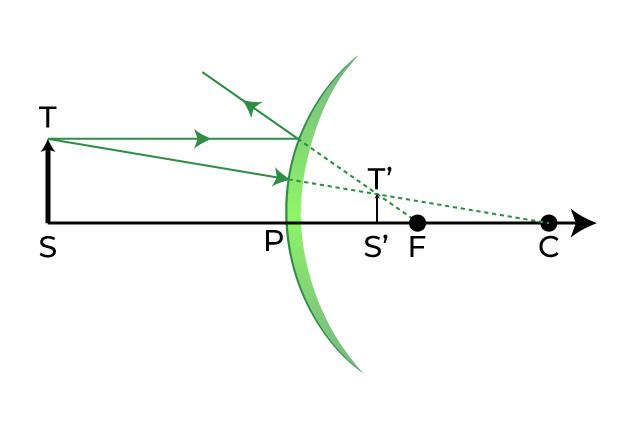Properties of image: The image formed is diminished in size as well as virtual and erect.

## Mirror Formula

To do the sums related to the spherical mirrors, the formula used is known as the mirror formula. It is used to calculate the focal length, image distance, object distance, and also magnification or any other thing required. We usually put the formula first and then put the signs so as to do the sums to minimize any error which can be generated.

The sign conventions which are to be followed while using the mirror formula are fixed so from the above-given diagram we can easily put the signs according to the requirement to get the required result.

The formula is given below,where

u = object distance,

v = image distance,

f = focal length of mirror

## Sign Conventions for Spherical Mirrors

• Usually, if the object is located on the left side of the principal axis from the mirror then the object distance is taken negatively.
• While if it is located on the right side it is taken to be positive.
• The sign of focal length depends on the type of mirror we are using, as for the concave mirror it is negative and for the convex mirror on the other hand is positive always.
• It is to be mentioned again that we have to follow the sign conventions strictly to get the correct answer.
• Heights which are above the principal axis are positive and below are negative.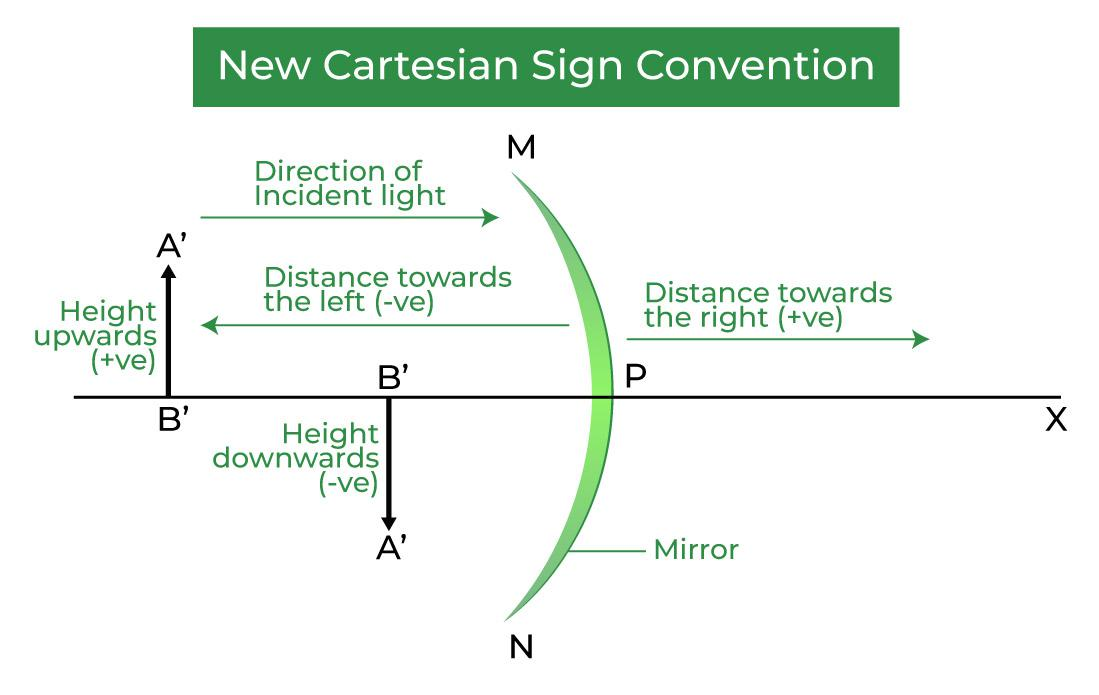## Solved Examples on Concave and Convex Mirrors

Example 1: What is the image distance in the case of a concave mirror if the object distance is 4 cm? It is given that the focal length of the mirror is 2 cm.

Solution:

As we know from mirror formula,Where u= object distance= -4cm

v= image distance=?

f= focal length of mirror= -2cm

Putting values we getHence the object is located 4 cm in front of the mirror.

Example 2: What is the image distance in the case of a concave mirror if the object distance is 32 cm? It is given that the focal length of the mirror is 16 cm. State the nature and the size of the image which is formed.

Solution:

As we know from mirror formula,where u = object distance= -32cm

v = image distance=?

f = focal length of mirror= -16cm

Putting values we getHence the object is located 8 cm in front of the mirror. And the image formed is real and inverted. As it is located at the centre of curvature hence the size of the image is also same as that of object.

Example 3: What is the image distance in the case of the convex mirror if the object distance is 12 cm? It is given that the focal length of the mirror is 12 cm.

Solution:

As we know from mirror formula,where u = object distance = -12 cm

v= image distance=?

f= focal length of mirror= 12cm

Putting values we get[Tex]\dfrac{1}{v} + \dfrac{1}{-12} = \dfrac{1}{12}\\\dfrac{1}{v} = \dfrac{1}{12}-\dfrac{1}{-12}\\ v = 6\text{ cm}[/Tex]

Hence the image is located 6cm behind the mirror. The nature of the image is virtual and erect and it is between the focus and the pole behind the mirror. The size of the image is found to be diminished.

Example 4: What is the image distance in the case of a concave mirror if the object distance is 10 cm? It is given that the focal length of the mirror is 10 cm.

Solution:

As we know from mirror formula,Where u= object distance= -10cm

v= image distance=?

f= focal length of mirror= -10cm

Putting values we getHence the image will be formed at infinity.

Example 5: What is the image distance in the case of a convex mirror if the object is at the focus of the mirror? It is given that the focal length of the mirror is 10 cm. What can you say about the nature of the image formed and also the size?

Solution:

As we know from mirror formula,Where u= object distance= -10cm

v= image distance=?

f= focal length of mirror= +10cm

Putting values we getHence the image is located 5 cm behind the mirror. The image formed is virtual and erect and size is diminished.

## FAQs on Concave and Convex Mirrors

### Q1: Which mirror is used by the dentist?

A concave mirror is used by the dentist. Because a concave mirror helps to see things more wider.

### Q2: What is another name for the concave mirror?

Due to the converging nature of the concave mirror, it can also be called a converging mirror.

### Q3: What are the applications of convex mirrors? State any two.

Solution:

Following are the two application of convex mirror:

1. As rear view mirrors in vehicles
2. As magnifying glass

### Q4: What is the difference between a concave mirror and a convex mirror?

The spherical mirrors in which the inner side is reflecting in nature are called concave mirrors. While the spherical mirrors in which the outer side is reflecting in nature are called convex mirrors.